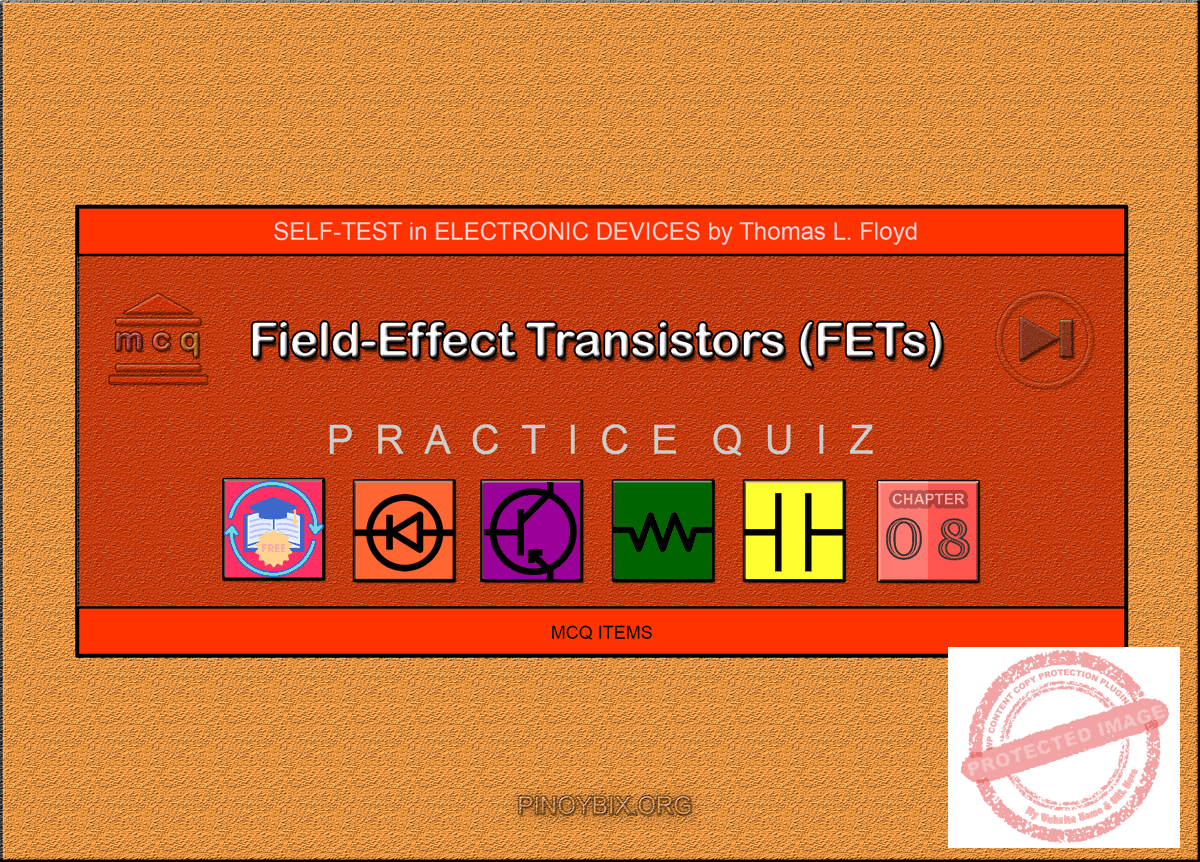# Floyd Self-test in Field-Effect Transistors (FETs)

(Last Updated On: December 5, 2019)This is the Self-test in Chapter 8: Field-Effect Transistors (FETs) from the book Electronic Devices Conventional Current Version, 9th edition by Thomas L. Floyd. If you are looking for a reviewer in Electronics Engineering this will definitely help you before taking the Board Exam.

#### Floyd Self-test Chapter 8 Topic Outline

• Floyd Self-test in Field-Effect Transistors (FETs)
• Floyd Self-test in JFET
• Floyd Self-test in JFET Characteristics and Parameters
• Floyd Self-test in JFET Biasing
• Floyd Self-test in The Ohmic Region
• Floyd Self-test in MOSFET
• Floyd Self-test in MOSFET Characteristics and Parameters
• Floyd Self-test in MOSFET Biasing
• Floyd Self-test in IGBT

If you are looking for the Multiple Choice Questions in Floyd’s Electronic Devices proceed to

#### Start Practice Exam Test Questions

Choose the letter of the best answer in each questions.

1. The JFET is

(a) a unipolar device

(b) a voltage-controlled device

(c) a current-controlled device

Solution:

2. The channel of a JFET is between the

(a) gate and drain

(b) drain and source

(c) gate and source

(d) input and output

Solution:

3. A JFET always operates with

(a) the gate-to-source pn junction reverse-biased

(b) the gate-to-source pn junction forward-biased

(c) the drain connected to ground

(d) the gate connected to the source

Solution:

4. For VGS = 0 V, the drain current becomes constant when VDS exceeds

(a) cutoff

(b) VDD

(c) VP

(d) 0 V

Solution:

5. The constant-current region of a FET lies between

(a) cutoff and saturation

(b) cutoff and pinch-off

(c) 0 and IDSS

(d) pinch-off and breakdown

Solution:

6. IDSS is

(a) the drain current with the source shorted

(b) the drain current at cutoff

(c) the maximum possible drain current

(d) the midpoint drain current

Solution:

7. Drain current in the constant-current region increases when

(a) the gate-to-source bias voltage decreases

(b) the gate-to-source bias voltage increases

(c) the drain-to-source voltage increases

(d) the drain-to-source voltage decreases

Solution:

8. In a certain FET circuit, VGS = 0 V, VDD = 15 V, IDSS = 15 mA, and RD = 470Ω. If RD is decreased to 330Ω, IDSS is

(a) 19.5 mA

(b) 10.5 mA

(c) 15 mA

(d) 1 mA

Solution:

9. At cutoff, the JFET channel is

(a) at its widest point

(b) completely closed by the depletion region

(c) extremely narrow

(d) reverse-biased

Solution:

10. A certain JFET datasheet gives The pinch-off voltage, VP,

(a) cannot be determined

(b) is –4V

(c) depends on VGS

(d) is +4V

Solution:

11. The JFET in Question 10

(a) is an n channel

(b) is a p channel

(c) can be either

Solution:

12. For a certain JFET, IGSS = 10 nA at VGS =10 V. The input resistance is

(a) 100 MΩ

(b) 1 MΩ

(c) 1000 MΩ

(d) 1000 mΩ

Solution:

13. For a certain p-channel JFET, VGS(off ) = 8 V. The value of VGS for an approximate midpoint bias is

(a) 4 V

(b) 0 V

(c) 1.25 V

(d) 2.34 V

Solution:

14. In a self-biased JFET, the gate is at

(a) a positive voltage

(b) 0 V

(c) a negative voltage

(d) ground

Solution:

15. The drain-to-source resistance in the ohmic region depends on

(a) VGS

(b) the Q-point values

(c) the slope of the curve at the Q-point

(d) all of these

Solution:

16. To be used as a variable resistor, a JFET must be

(a) an n-channel device

(b) a p-channel device

(c) biased in the ohmic region

(d) biased in saturation

Solution:

17. When a JFET is biased at the origin, the ac channel resistance is determined by

(a) the Q-point values

(b) VGS

(c) the transconductance

Solution:

18. A MOSFET differs from a JFET mainly because

(a) of the power rating

(b) the MOSFET has two gates

(c) the JFET has a pn junction

(d) MOSFETs do not have a physical channel

Solution:

19. A D-MOSFET operates in

(a) the depletion mode only

(b) the enhancement mode only

(c) the ohmic region only

(d) both the depletion and enhancement modes

Solution:

20. An n-channel D-MOSFET with a positive VGS is operating in

(a) the depletion mode

(b) the enhancement mode

(c) cutoff

(d) saturation

Solution:

21. A certain p-channel E-MOSFET has a If VGS = 0 V, the drain current is

(a) 0 A

(b) ID(on)

(c) maximum

(d) IDSS

Solution:

22. In an E-MOSFET, there is no drain current until VGS

(a) reaches VGS(th)

(b) is positive

(c) is negative

(d) equals 0 V

Solution:

23. All MOS devices are subject to damage from

(a) excessive heat

(b) electrostatic discharge

(c) excessive voltage

(d) all of these

Solution:

24. A certain D-MOSFET is biased at VGS = 0 V. Its datasheet specifies IDSS= 20 mA and the value of the drain current

(a) is 0 A

(b) cannot be determined

(c) is 20 mA

Solution:

25. An IGBT is generally used in

(a) low-power applications

(b) rf applications

(c) high-voltage applications

(d) low-current applications

Solution:

### Complete List of Floyd Self-test in Electronic Devices

P inoyBIX educates thousands of reviewers and students a day in preparation for their board examinations. Also provides professionals with materials for their lectures and practice exams. Help me go forward with the same spirit.

“Will you subscribe today via YOUTUBE?”

Subscribe

PinoyBIX Engineering. © 2014-2020 All Rights Reserved | How to Donate? |